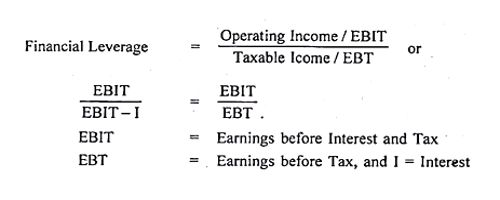The degree of financial leverage is a financial ratio that measures the sensitivity in fluctuations of a company’s overall profitability to the volatility of its operating income caused by changes in its capital structure. It is the relationship between percentage change in earnings per share and the percentage change in earnings before interest and tax (EBIT). It can also be determined by the relationship between EBIT and EBT (earnings before tax). If the Degree of Financial Leverage is high, the Earnings Per Share or EPS would be more unpredictable while all other factors would remain the same.

A degree of financial leverage is a leverage ratio that measures the sensitivity of a company’s earnings per share (EPS) to fluctuations in its operating income, as a result of changes in its capital structure.

It measures the percentage change in earnings per share (EPS) due to a percentage change in EBIT. It measures the percentage change in EPS for a unit change in operating income, also known as earnings before interest and taxes (EBIT). The financial leverage can be determined as given below.Calculation of DFL on the Basis of Income Statement:

DFL = EBIT/Ebit-I = EBIT/EBT

Calculation of DFL By Using Formula:

DFL = (Sales-variable cost- fixed cost/sales – variable cost-fixed cost-interest)

= S-VC-FC/S-VC-FC-I

Calculation of DFL by Using Percentage Change Method:

DFL = % change in EPS/% change in EBIT

Where,

DFL = degree of financial leverage

EBIT = earning before interest and tax

EBT = earning before tax

EPS = earning per share

I = Interest

This formula can be even used to compare data of many companies that can help an investor in deciding which company to invest in, based on the result of how much risk is attached with each companies capital structure. This ratio indicates that the higher the degree of financial leverage, the more volatile earnings will be. Since interest is usually a fixed expense, leverage magnifies returns, and EPS. This is good when operating income is rising, but it can be a problem when operating income is under pressure.

Applications

The use of financial leverage varies greatly by industry and by the business sector. It is one of the methods used to quantify a company’s financial risk (the risk associated with how the company finances its operations). There are many industry sectors in which companies operate with a high degree of financial leverage. It is useful for modeling what may happen to the net income of a business in the future, based on changes in its operating income, interest rates, and/or amount of debt burden. Retail stores, airlines, grocery stores, utility companies, and banking institutions are classic examples.

Information Source: